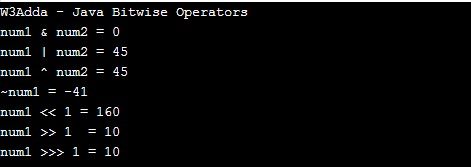# Java Bitwise Operators

In this tutorial you will learn about the Java Bitwise Operators and its application with practical example.

## Java Bitwise Operators

Bitwise operators are used to performing the bit-level operations over their operand.

Let A = 60; and B = 13;

Binary equivalent

A = 0011 1100

B = 0000 1101

Operator Meaning Example Description
& Binary AND (A & B) It returns 12 which is 0000 1100
| Binary OR (A | B) It returns 12 which is 0000 1100
^ Binary XOR (A ^ B) It returns 49 which is 0011 0001
~ One’s Complement (~A ) It returns -60 which is 1100 0011
<< shift left A << 2 It returns 240 which is 1111 0000
>> shift right A >> 2 It returns 15 which is 0000 1111

Example:-

When you run the above java program, you will see the following output.

Output:-In this tutorial we have learn about the Java Bitwise Operators and its application with practical example. I hope you will like this tutorial.